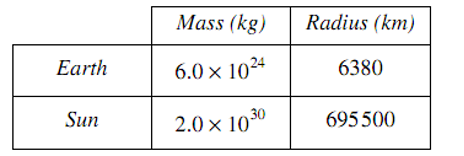# M5-S10: Kepler's Laws of Planetary Motion

• ### Investigate the relationship of Kepler’s Laws of Planetary Motion to the forces acting on, and the total energy of, planets in circular and non-circular orbits using: (ACSPH101)

• v=(2pir)/T
• (r^3)/(T^2)=(GM)/(4pi^2)

Kepler, without using a telescope, devised three main laws regarding planetary motion:

1. Planets orbit the Sun in an elliptical path, with the Sun being one of its foci
2. Imaginary line drawn from the planet to the Sun sweeps out equal area in equal time
3. Cube of semi-major radius of orbit is directly proportional to the square of the planet’s orbital period

For HSC physics, we model Kepler’s law using circles instead of ellipses. It is undeniably less accurate and correct but by doing this we can better consolidate the idea of ‘uniform circular motion in space’ with Kepler’s law of planetary motion.If the orbit is circular, the total distance/length of orbit equals to the circumference and thus, the tangential velocity (constant during uniform circular motion) equals to:

v=(2pir)/T

Recall when a planet is orbiting in uniform circular motion, it is required to travel at the orbital velocity given by the equation:

v_(orbital)=sqrt((GM)/r)

By equating the two equations above, we get:

(2pir)/T=sqrt((GM)/r)

(4pi^2r^2)/(T^2)=(GM)/r

(r^3)/(T^2)=(GM)/(4pi^2)

Therefore, by incorporating Newton’s Universal Law of Gravitation (which was used to prove orbital velocity), we are able to find the constant of proportionality outlined in Kepler’s third law.

Planets which orbit the same central object e.g. the Sun, regardless of their orbital path or period, the constant of proportionality is the same for all planets. This is particularly useful in finding orbital characteristics of a planet using values of another planet.

Practice Question 1

Assume the orbital period of Earth around the Sun is exactly 365 days.Use the data from the table to determine the average distance between Earth and the Sun (2 marks)

Practice Question 2

In a faraway galaxy, planets A and B orbit the same star.

 Orbital radius (km) Orbital period (hours) Planet A 3 x 107 7.5 Planet B 2 x 107 Unknown

Use the data from the table to determine the orbital period of planet B. (2 marks)

Practice Question 3

Planets X and Y orbit the same star of mass M. If planet X’s orbital period is eight times longer than that of planet Y, its orbital radius is:

1. Twice as much as planet Y
2. Four times as much as planet Y
3. Half as much as planet Y
4. Cannot be determined due to insufficient information

Previous section: Newton's Law of Gravitation